## Python Tutorial for Absolute Beginners

Python is one of the most widely used languages out there. Be it web development, machine learning and AI, or even micro-controller programming, Python has found its place just about everywhere.

This article provides a brief introduction to Python for beginners to the language. The article is aimed at absolute beginners with no previous Python experience, although some previous programming knowledge will help, but is not necessarily required.

I've found that the best way to learn is to try to understand the theory and then implement the example on your own. Remember, you will not get better at programming unless you practice it!

The article is divided into following sections:

### Why Learn Python

The question arises here that why you should learn Python. There are lots of other programming languages; you might have even learned some of them. Then why Python, what is so special about it? There are various reasons to learn Python, most important of which have been enlisted below.

• Easy to Learn

Python is considered one of the most beginner-friendly languages. The syntax of Python is the simplest of all. You don't have to learn complex variable types, use of brackets for grouping code blocks and so on. Python is built upon the fundamental principle of beginner-friendliness.

• Highly In-Demand

According to a recent survey by indeed.com, Python developers are the second highest paid developers in USA. The huge job potential of Python can be estimated by the fact that in 2014 the average hiring rate for programmers decreased by 5% but Python developers still saw an increase of 8.7%.

• Ideal for Web development

Python is lightning fast when compared with other web development languages such as PHP and ASP.NET. Also, Python has myriad of amazing frameworks such as Django, Flask, and Pylons, which makes web development even simpler. Websites like Instagram, Pinterest and The Guardian are all based on the popular Django framework.

• Used Heavily for Machine learning and AI

Python is the most widely used language for machine learning and artificial intelligence operations. Python libraries such as TensorFlow and scikit-learn makes AI tasks much simpler when compared to MATLAB or R, which previously was the most widely used environment for data science and AI tasks.

• Works with Raspberry Pi

Python is the most popular programming language for the Raspberry Pi, which is a pocket size microcomputer used in a wide range of applications such as robots, gaming consoles, toys. In short, learn Python if you want to build things with the Raspberry Pi.

• Corporate Darling

It would not be an exaggeration if we say that Python is the darling of all all the big corporate companies such as google, yahoo, NASA, Disney, IBM etc. These companies have incorporated Python at the core of many of its applications.

• Large Community

Python has one of the largest programming communities online and it continues to grow. Python has the fifth largest Stack Overflow community, and third largest meet-up community. And most importantly, it is the 4th most used language at GitHub, which means there is tons of existing code to learn from.

### Installation and Setup

Though there are several ways to install Python for Windows, but for the sake of this article we will use Anaconda. It is undoubtedly the most widely used Python environment at the moment. To download Anaconda, go to this link:

Scroll down a bit and you should see the download options. Select, Python 3.6 as shown in the following screenshot: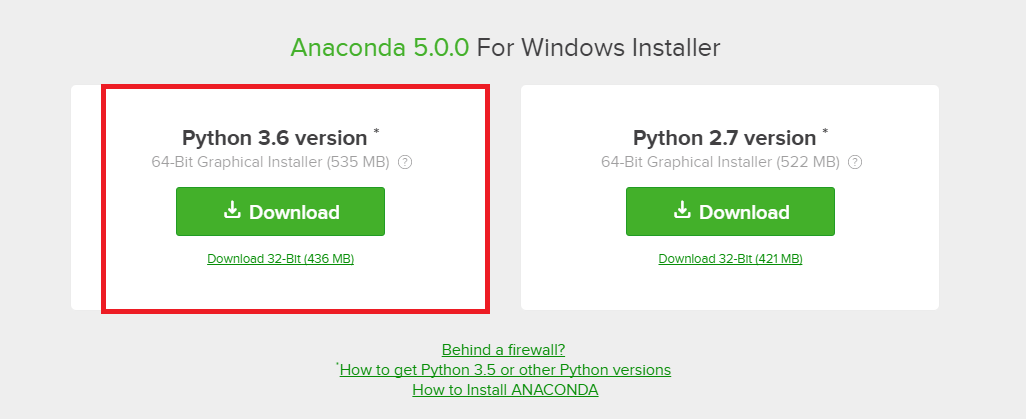This will download an Anaconda installer to your computer. Open the installer and you will see the following options: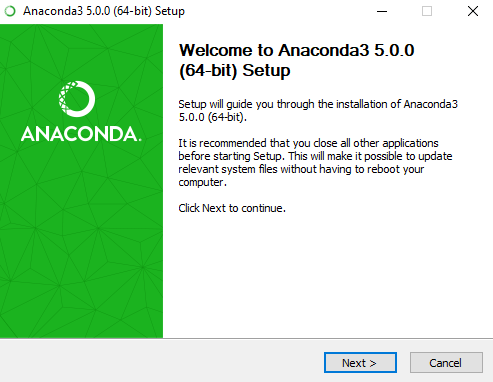• Click the "Next" button. Terms and Condition will appear, you can read if you have enough time but you can click "I Agree" anyways.
• In the next window select the type of installation you want. If you are absolute beginner to Python I would recommend selecting, "Just me" option.
• Next, select the installation folder (Default is best).
• Advance options dialogue box will appear, keep the first option unchecked and the second checked and click "Install". This is shown in the following screenshot.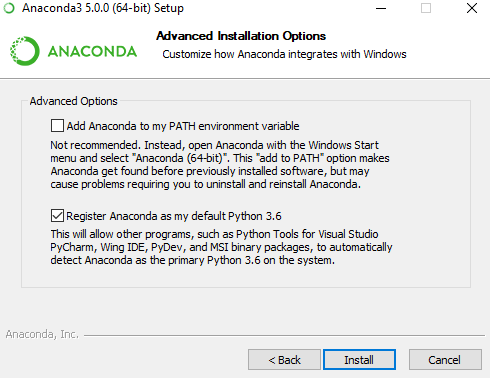Now sit back and have some coffee, the installation might take some time.

Once the installation is complete, you will see the message: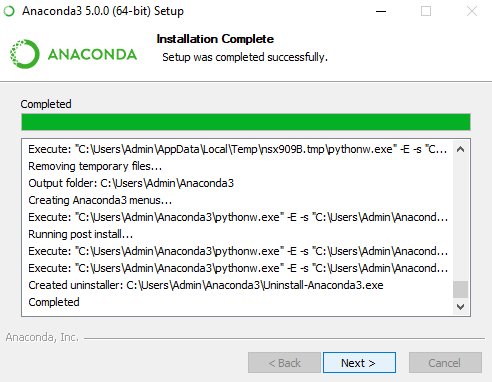Click "Next" and then "Finish" button on the subsequent dialogue box to complete the installation.

Although you can run Python programs via command line as well, it is typically better for beginners to use a text editor. Luckily, with the installation of Anaconda, you get the Jupyter Notebook installed as well. The "Jupyter Notebook" is a cloud based application that allows users to create, share, and manage their documents. We will use Jupyter to write our Python code in this article.

To open Jupyter, you can go to Start Menu and find the "Jupyter Notebook" application. You can also search for it in Applications. This is shown in the following:Open the "Jupyter Notebook" application. It will then be opened in your default browser. For compatibility, I would recommend that you use Google Chrome as your default browser, but other browser types like Firefox would work as well.

When the application opens in your browser, you will see the following page: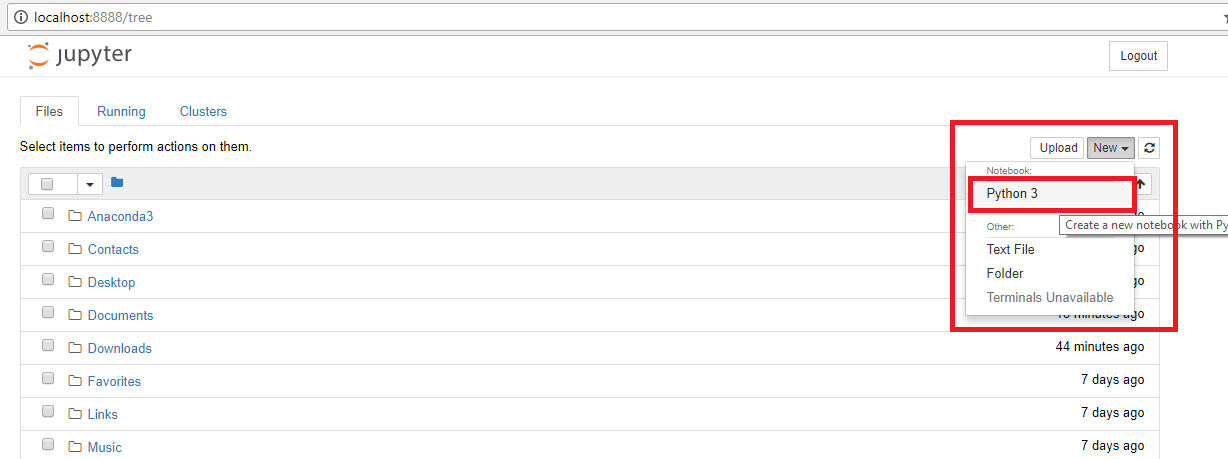On the right hand side of the page, you will see an option "New". Click that buttonand a dropdown list will appear. Select, "Python 3" from the dropdown list. This will open a brand new notebook for you, which looks like this:Here you can easily write, save, and share your Python code.

Let's test and make sure everything is working fine. To do this, we'll create a simple program that prints a string to the screen.

Enter the following code in the text field in your Jupyter notebook (shown in the screenshot above):

print("Welcome to Python!")


The print does exactly what it sounds like, it simply prints some text to the screen. The text you want to display is entered within the double quotations inside the parenthesis that follow the print keyword.

To run code in "Jupyter Notebook" just press "Ctrl + Enter". The output of the above code should look like the following: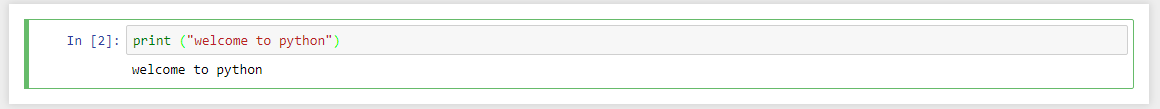And there you have it, we have successfully executed our first Python program! In the following sections, we'll continue to use Jupyter to teach and discuss some core Python features, starting with variables.

### Python Variables

Simply put, variables are memory locations that store some data. You can use variables to store a value, whether it be a number, text, or a boolean (true/false) value. When you need to use that value again later in your code, you can simply use the variable that holds that value. You can almost think of them as simple containers that store things for you for later use.

It is important to mention here that unlike Java, C++ and C#, Python is not a strongly typed language. This means that you do not need to specify the type of variable according to the value it holds. Python implicitly decodes the variable type at runtime depending upon the type of data stored in it. For instance you don't need to specify int n = 10 to define an integer variable named "n". In Python we simply write n = 10 and the type of variable "n" will be implicitly understood at runtime.

There are five different core data types in Python:

• Numbers
• Strings
• List
• Tuples
• Dictionaries

In this section we will only take a look at numbers and strings. Lists, tuples, and dictionaries will be explained further in their own respective section later in this article.

#### Numbers

The number type of variables store numeric data. Take a look at the following simple example:

num1 = 2
num2 = 4
result = num1 + num2
print(result)


Here in the above example we have two numeric variables, num1 and num2, with both containing some numeric data. There is a third number type variable, result, which contains the result of the addition of the values stored in num1 and num2 variables. Finally, on the last line the result variable is printed to the screen.

The output will be as follows: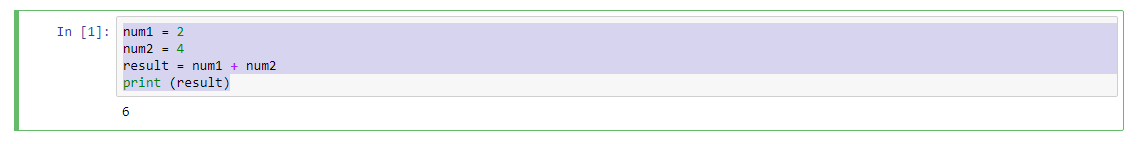There are four different number data types in Python:

• Integers, such as real whole-valued numbers: 10
• Long integers, which have "L" at the end for values: 1024658L
• These can also be used in hexadecimal and octal form
• Floating point data, which are numbers expressed in decimals: 3.14159
• Complex data, which is used to represent complex number types: 2 + 3j

#### Strings

Strings are used to store text data in Python. Take a look at the following example:

fname = "Adam"
sname = " Grey"
fullname = fname + sname

print(fullname)


In the above example we have two string variables: fname and sname. These store the first name and surname of some person. To combine these two strings we can use "+" operator in Python. Here we are joining the fname and sname variables and store the resultant string in the fullname variable. Then we print the fullname variable to the screen.

The output is as follows:There are hundreds of strings operations in Python, we will have a dedicated article on these functions in future.

### Operators in Python

Operators in programming are the constructs that allow you to manipulate an operand to perform a specific function. They are very similar to real life operators, such as arithmetic operators e.g addition, subtraction, greater than, less than, and AND/OR operators, etc.

There are seven types of operators in Python:

• Arithmetic Operators
• Logical Operators
• Assignment Operators
• Comparison Operators
• Bitwise Operators
• Identity Operators
• Member Operators

In this article we'll keep it simple and study only the first four operators. The other operators are beyond the scope of this article.

#### Arithmetic Operators

Arithmetic operators perform mathematical operations such as addition, subtraction, multiplication, division, and exponential functions on the operands. The detail of arithmetic functions have been given in the following table:

Suppose the variables n1 and n2 have values of 4 and 2, respectively.

Operator Functionality Example
Addition (+) Adds two or more operands n1 + n2 = 6
Subtraction (-) Subtracts second operand from the first n1 - n2 = 2
Multiplication (*) Multiply two or more operands n1 * n2 = 8
Division (/) Divide the first operand by second n1 / n2 = 2
Modulus (%) Finds remainder when the first operand is divided by second n1 % n2 = 0
Exponent (**) Takes the power of first operand to the second n1 ** n2 = 16

You may recall seeing an example of the arithmetic addition operator earlier in the Number data variable section. In Python, addition operators can apply to any kind of number, and even strings.#### Logical Operators

The logical operators, which help you perform simple Boolean algebra, supported by Python are as follows:

Suppose o1 and o2 have values True and False, respectively.

Operator Functionality Example
AND Returns true of the all the conditions are true (o1 and o2) is false
OR Returns true if any of the condition is true (o1 or o2) is true
NOT Return the reverse of the actual logical state Not(o1) is false

The following code helps explain the above operators with an example:

o1 = True
o2 = False
r1 = (o1 and o2)
print(r1)

r2 = (o1 or o2)
print(r2)

r3 = not(o1)
print(r3)


The output of the above code is:

False
True
False


#### Assignment Operators

Assignment operators allow you to "give" a value to variables, which may be the result of an operation. The following table contains some of the most widely used assignment operators in Python:

Operator Functionality Example
Assign Assign the value on the right to the variable on the left r = n1 + n2 assigns value of n1 + n2 into r
Add and Assign Adds both the operands and assign the result to the variable on the left one n1 += n2 is the same as n1 = n1 + n2
Subtract and Assign Subtracts right operand from the left and assign the result to the left n1 -= n2 means n1 = n1 - n2
Multiply and Assign Multiplies both the operands and assign the result to the left one n1 *= n2 means n1 = n1 * n2
Divide and Assign Divides both the operands and assign the result to the left one n1 /= n2 means n1 = n1 / n2
Modulus and Assign Take modulus by dividing left operand by the right and assign the result to the left one n1 %= n2 means n1 = n1 % n2
Exponent and Assign Take exponent of right operand to the left and assign the value to left n1 **= n2 means n1 = n1 ** n2

Take a look at the following example to see some of the assignment operators in action:

n1 = 4
n2 = 2

n1 += n2
print(n1)
n1 = 4

n1 -= n2
print(n1)
n1 = 4

n1 *= n2
print(n1)
n1 = 4

n1 /= n2
print(n1)


The output of the above code will be:

6
2
8
2.0


Notice how in the last operation we get a floating point number as our result, whereas we get integer numberes in all of the prevoius operations. This is because this is the only mathematical operation in our example that could turn two integer numbers in to a floating point number.

#### Comparison Operators

Comparison operators are used to compare two or more operands. Python supports the following comparison operators:

Suppose n1 is 10 and n2 is 5 in the following table.

Operator Functionality Example
== Returns True if the two operands are equal to each other (n1 == n2) is not true
!= Returns true of two operands are not equal (n1 != n2) is true
> Returns true if left operand is greater than right operand (n1 > n2) is true
< Returns true if left operand is smaller than right operand (n1 < n2) is not true
>= Returns true if left operand is equal to or greater than the right operand (n1 >= n2) is true
<= Returns true if left operand is equal to or smaller than the right operand (n1 =< n2) is not true

Consider the following simple example of comparison operator:

n1 = 10
n2 = 5

print(n1 == n2)
print(n1 != n2)
print(n1 > n2)
print(n1 < n2)
print(n1 >= n2)
print(n1 <= n2)


The output of the above code is:

False
True
True
False
True
False


### Conditional Statements

Conditional statements are used to select the code block that you want to execute based upon a certain condition. Suppose in a hospital management system, you want to implement a check that the patient with age over 65 can receive priority treatment while the others cannot, you can do so with conditional statements.

There are four types of conditional statements:

• "if" statements
• "if/else" statements
• "if/elif" statement
• Nested "if/else" statements

Basically, the second and third types are just extensions of the first statement type.

#### If Statement

The "if statement" is the simplest of all the statements. If the given condition resolves to true (like 1 < 10), then the code block that follows the "if statement" is executed. If the condition returns false (like 1 > 10), then the code is not executed.

Take a look at the following example.

age = 67

if age >= 65:
print("You are eligible for priority treatment.")



Pay close attention to the syntax of conditional statements. In most of the other programming languages, the code block that is to be executed if the "if" condition returns true is enclosed inside brackets. Here in Python you have to use colon after the "if" condition and then you have to indent the code that you want to execute if the condition returns true.

Python is widely considered to be a much cleaner language than many others because of the absence of brackets. Indentation is used instead to specify scope, which has its own pros and cons.

In the above example we have an age variable with value 67. We check if age is greater than 65, and if this condition returns true then we print a message telling the user that he/she is eligible for priority treatment. Notice that this message is indented, which tells us it is the code to be executed following a true condition. Finally, we simply print the thank you message on the screen. The output of this code will be:

You are eligible for priority treatment.


Now let's set the value of the age variable to 55 and see the difference.

age = 55

if age >=65:
print("You are eligible for priority treatement.")


The output of the above looks like this:

Thank you for your visit


Notice that this time the condition did not return true, hence the statement telling the patient that he is eligible for priority treatment is not printed to the screen. Only greetings have appeared since they were not inside (indented) the body of the "if" statement.

#### If/Else Statement

The "if/else" statement is used to specify the alternative path of execution in case the "if" statement returns false. Take a look at the following example:

age = 55

if age >=65:
print("You are eligible for priority treatment.")
else:
print("You are eligible for normal treatment")



Here the code block followed by the "else" statement will be executed since the age variable is 55 and the "if" condition will return false. Hence, the "else" statement will be executed instead. The output will be as follows:

You are eligible for normal treatment


#### If/Elif Statment

The "if/elif" statement is used to implement multiple conditions. Take a look at the following example:

age = 10

if age >= 65:
print("You are eligible for priority treatment.")
elif age > 18 and age < 65:
print("You are eligible for normal treatment")
elif age < 18:
print("You are eligible for juvenile treatment")



In the above code we have implemented three conditions. If age is greater than 65, if age is between 65 and 18, and if the age is less than 18. Based on the value of the age, different print statement will be executed. Here since the age is 10, the second conditional returns true and you will see the following output:

You are eligible for juvenile treatment


If none of the conditionals were to return true then none of the print() statements would have executed. This differs from the "if/else" example where either "if" is executed or "else" is executed. In the case of "if/elif", this isn't necessarily the case. However, you can add a normal "else" statement at the end that gets executed if none of the conditions above it return true.

Using this method I just described, we could re-write the previous example to look like this:

age = 10

if age >= 65:
print("You are eligible for priority treatment.")
elif age > 18 and age < 65:
print("You are eligible for normal treatment")
else:
print("You are eligible for juvenile treatment")



This code would result in the same output as the previous example.

#### Nested If Else Statement

Nested "if/else" statements are used to implement nested conditions (i.e. conditions within another condition). Consider the following example:

age = 67
insurance = "yes"

if age >= 65:
print("You are eligible for priority treatment.")
if insurance == "yes":
print("The insurance company will pay for you.")
else:
print("You have to pay in advance.")
else:
print("You are eligble for normal treatment")



Here we have an outer condition that if age is greater than or equal to 65, then check that if patient has insurance or not. If the patient has insurance, the insurance company will pay the bill later, otherwise the patient has to pay in advance.

### Loops

Iteration statements, or more commonly known as loops, are used to repeatedly execute a piece of code multiple times. Consider if you have to print names of 100 persons on the screen. You will either have to write 100 print statements or you will have to use hundreds of escape characters in one print statements. If you have to perform this task repeatedly you have to write hundreds of thousands of tedious lines of code. A better way is to make use of loops.

There are two main types of loops in Python:

• For loop
• While Loop

Keep in mind that you can nest loops just like we did with the conditional statements, but we won't go in to that here.

#### The For Loop

The "for loop" is used to iterate over a collection of elements. The loop keeps executing until all the elements in the collection have been traversed. Take a look at the simple example of for loop:

nums = [1, 2, 4, 5, 6, 7, 8, 9, 10]

for n in nums:
print(5 * n)


The above example simply prints the product of each item in nums and 5. Here we have a list nums which contains integers from 1 to 10. Don't worry, we will study lists in detail in a later section. For now, just consider it as a collection of elements, which in this case are numbers.

Pay close attention to the code above. It follows following syntax:

for [temp_var] in [collection]:
[statements]


In the first iteration of the "for loop" the 1 is stored in the temporary variable n. This 1 is multiplied by 5 and the result is printed on the screen. In the second iteration the second element from the nums collection (i.e. 2) is stored in the n variable and 2 is multiplied by 5. These iterations continue until all the elements in the nums collection have been traversed. After the last element (10) is encountered, the loop stops and code execution moves past the "for loop".

The output of the above code is:

5
10
20
25
30
35
40
45
50


#### The While Loop

The "while loop" is different from the "for loop" in that it keeps executing while a certain condition keeps returning true. After each iteration of the while loop, the condition is re-evaluated. When the condition finally returns false, the while loop stops executing and exits.

Take a look at the following example:

x = 50

while x > 0:
print(x)
x = x - 5


Here the loop will keep executing until the value of x becomes negative. The x variable has initially value of 50 and during each iteration we decrement it by 5. So, after 10 iterations the value will become negative and the loop will then stop executing.

The output will look like this:

50
45
40
35
30
25
20
15
10
5


While loops are good for times when you don't already know how many iterations you need. For loops iterate a set number of times, whereas while loops can iterate an unknown number of times, or even an infinite number of times.

### Functions in Python

Functions in programming are constructs that perform specific tasks. Functions come handy in scenarios when you have to perform a task multiple times throughout your code. Instead of re-writing the same functionality again and again, instead you can create a function that performs that task and then call that function wherever and whenever you want.

Notice that there is a difference between doing a task repeatedly and doing a task multiple times. Loops are used where you have to perform a task repeatedly in sequence. Functions, on the other hand, are used when you have to perform the same task at different places throughout your code.

Consider a scenario where you have to print a long statement to screen at different times. Instead, write a function that prints the statement you want and then call the function wherever you want to print the statement.

Take a look at the following example:

def displayWelcome():
print("Welcome to Python. This article explains the basics of Python for absolute beginners!")
return;

displayWelcome()
print("Do something here")
displayWelcome()
print("Do some other stuff here")


There are two things I'd like to point out in this code: the function definition and the function calls.

Function definition refers to defining the task performed by the function. To define a function you have to use keyword def followed by the name of the function, which is displayWelcome in the above example. You can use any function name, but to use semantic function. The function name is followed by opening and closing parenthesis. The parenthesis are used to define parameters or any default input values, which we will see this in next example. After the parenthesis you have to use colon and on the next line the body of the function is defined. A function usually ends with a return statement, but it is not required if a value is not being returned.

In the second part of our example code you'll see the function call. To call a function you simply have to write the function name followed by pair of parenthesis. If a function accepts parameters, you have to pass them inside parenthesis.

The output of the above code will be:

Welcome to Python. This article explains the basics of Python for absolute beginners
Do something here
Welcome to Python. This article explains the basics of Python for absolute beginners
Do some other stuff here


You can see that our long string was printed twice. Once before the "Do something here" statement, and once after it, which matches the order of our function calls within the code.

You can imagine how important this is to programming. What if we needed to perform a more complex task like downloading a file or performing a complex calculation? It would be wasteful to write out the full code multiple times, which is where functions come in to play.

#### Functions with Parameters

Now let's see how to pass parameters to a function. A parameter is just a variable that is given to the function from the caller.

Let's write a function that adds two numbers passed to it as parameters in the parenthesis:

def addNumbers(n1, n2):
r = n1 + n2
return r;

print(result)

print(result)

print(result)


In the above code we have the addNumbers function, which accepts two values from the function call. The values are stored in the n1 and n2 variables. Inside the function these values are added and stored in the r variable. The value in the r variable is then returned to the caller of the function.

In the first call to addNumbers we pass two values, 10 and 20. Note that the order of parameters matter. The first value in the function call is stored in the first parameter in the function, and the second value is stored in the second parameter. Therefore 10 will be stored in n1 and 20 will be stored in n2. We then display the result of the function via the print statement. This function is called a total of three times, each time with different parameter values.

The result of the above code will be:

30
100
40


You can see that every time the function is called, our result variable contains the addition of the two numbers passed.

### Lists, Tuples, and Dictionaries

Lists, tuples, and dictionaries are three of the most commonly used data structures in programming. Though all of them store a collection of data, the main difference lies in the following:

• How you place data in the data structure
• How the data is stored within the structure
• How data is accessed from the data structure

In the next few sections you'll see some of these properties for each data structure.

#### Lists

Lists are used to store a collection of items of varying data types. The elements are stored inside square brackets where each element is separated from each other with a comma.

Let's see how to create a simple list:

randomlist = ['apple', 'banana', True, 10, 'Mango']


You can see we have stored strings, a number, and a Boolean in this list. In Python (unlike other strongly typed languages), a list can store any type of data in a single list, as shown above. More commonly, however, lists tend to store many different values of the same data type.

##### Accessing List Elements

To access an element in a list simply write the name of the list variable followed by pair of square brackets. Inside the brackets specify the index number of the element you want to access. It is important to note that lists in Python (and many other programming languages), list indexes start at 0. This means the first element in every list is at position 0, and the last element is at position n-1, where n is the length of the list. This is called zero-based indexing.

Take a look at this code:

print(randomlist)
print(randomlist)


Here we are accessing the first and fifth element of the randomlist list. The output will be:

apple
Mango


You may have also noticed that the elements in the list remain in the order in which they are stored. They will remain in the same order unless they are explicitly moved or they are removed.

##### Assigning New List Elements

To assign a value to an existing list position, you must specify the index of the position you want to assign the value to and then use the assignment operator (=) to actually assign the value.

See the code below:

# Define the list
randomlist = ['apple', 'banana', True, '10', 'Mango']

# Print the current value at index 0
print(randomlist)

# Assign a new value at index 0
randomlist = 'Peach'

# Print the updated value
print(randomlist)


Here we have updated the first element of the list. We displayed the value of the element before and after the update to show the change.

In the last sub-section we showed how to assign a value to a list, but this only applies if an item already exists at that position. What if we wnat to expand the size of the list and add a new item without getting rid of any of our previous items? We do this by using the append() function.

randomlist = ['apple', 'banana', True, '10', 'Mango']

print(randomlist)

randomlist.append(0)

print(randomlist)


When running this code you will notice that the value 0 is shown at the end of the list after calling the append function. Our list now has a total of 6 elements in it, including our new value.

##### Deleting List Elements

To remove an element, we simply use the del keyword. Take a look at the following example to see how it is used:

randomlist = ['apple', 'banana', True, '10', 'Mango']

print(randomlist)

# Remove the second element
del randomlist

print(randomlist)


Here we deleted the second element of the randomlist list. We use the print statement to show the list before and after deleting the element. The output will be as follows:

['apple', 'banana', True, '10', 'Mango']
['apple', True, '10', 'Mango']


#### Tuples

Tuples are similar to list in that they store elements of varying data types. The main distinction between tuples and lists is that tuples are immutable. This means that once you have created a tuple you cannot update the value of any element in the tuple, nor can you delete an element.

In terms of syntax, tuples differ from lists in that they use parenthasis, whereas lists use square brackets. Even with all of these differences, tuples are still very similar to lists. Elements are accessed the same, and element order is preserved, just like lists.

Here is how you can create a tuple:

randomtuple = ('apple', 'banana', True, '10', 'Mango')

##### Accessing Tuple Elements

Tuple elements can be accessed in same way as lists:

randomtuple = ('apple', 'banana', True, '10', 'Mango')

print(randomtuple)
print(randomtuple)


In the above script we are accessing the second and fifth element of the tuple. As expected, this would result in the following output:

banana
Mango

##### Assigning Values to Tuple Elements

As discussed earlier, it is not possible to assign new values to already declared tuple elements. So you cannot do something like this:

randomtuple = 10     # This operation is not allowed


Attempting an assignment like this results in the following error being raised:

TypeError: 'tuple' object does not support item assignment

##### Deleting a Tuple Element

You cannot delete an individual tuple element. Attempting to do so would result in a raised error, just like we showed when you try to re-assign an element:

TypeError: 'tuple' object doesn't support item deletion


However you can delete a tuple itself using "del" function as shown in the following example:

randomtuple = ('apple', 'banana', True, '10', 'Mango')

print(randomtuple)

del randomtuple

print(randomtuple)


If you try to access a deleted tuple, as in the second print statement above, you will receive the following error message:

NameError: name 'randomtuple' is not defined


#### Dictionaries

Like lists and tuples, dictionary data structures store a collection of elements. However, they differ quite a bit from tuples and lists because they are key-value stores. This means that you give each value a key (most commonly a string or integer) that can be used to access the element at a later time. When you have a large amount of data, this is more efficient for accessing data than traversing an entire list to find your element.

When you create a dictionary, each key-value pair is separated from the other by a comma, and all of the elements are stored inside curly brackets. See the following code:

randomdict = {'Make': 'Honda', 'Model': 'Civic', 'Year': 2010, 'Color': 'Black'}


Dictionaries are very useful when you have a lot of information about a particular thing, like the car example we showed above. They're also useful when you need to access random elements in the collection and don't want to traverse a huge list to access them.

##### Accessing Dictionary Elements

Dictionary elements are accessed using their keys. For instance if you want to access the first element, you will have to use its key, which in this case is 'Make'. Take a look at the following example to see the syntax:

randomdict = {'Make': 'Honda', 'Model': 'Civic', 'Year': 2010, 'Color': 'Black'}

print(randomdict['Make'])
print(randomdict['Model'])


Here we are accessing the first and second elements of the randomdict dictionary via their keys. The output will look like this:

Honda
Civic


Because dictionary elements are accessed using their keys, the elements are not ordered in the data structure, and it is not as straight-forward to iterate over like lists are.

##### Assigning Values to Dictionary Elements

To assign value to already existing dictionary element you first have to access the element and then assign a new value to it. The following example shows this:

randomdict = {'Make': 'Honda', 'Model': 'Civic', 'Year': 2010, 'Color': 'Black'}

print(randomdict['Make'])
randomdict['Make'] = 'Audi'
print(randomdict['Make'])


The output will have this:

Honda
Audi

##### Deleting Dictionary Elements

There are three different ways to delete elements in dictionaries: You can delete individual elements, you can delete all the elements, or you can delete the entire dictionary itself. The following example shows all of these three ways:

randomdict = {'Make': 'Honda', 'Model': 'Civic', 'Year': 2010, 'Color': 'Black'}

# Displaying complete dictionary
print(randomdict)

# Deleting one element
del randomdict['Make']
print(randomdict)

# Clearing whole dictionary
randomdict.clear()
print(randomdict)

# Deleting dictionary itself
del randomdict
print(randomdict)


Here we are displaying the dictionary after performing each of the three delete operations. Don't worry about the "#" and proceeding text in the code - these are there to make comments about the code. Comments are not executed, they just provide information about the code, and are purely optional.

The output of the above code will be:

{'Color': 'Black', 'Make': 'Honda', 'Model': 'Civic', 'Year': 2010}
{'Color': 'Black', 'Model': 'Civic', 'Year': 2010}
{}
Traceback (most recent call last):
File "dict_test.py", line 16, in <module>
print(randomdict)
NameError: name 'randomdict' is not defined


Notice that since we deleted the dictionary at the end, therefore an error is thrown indicating that randomdict is not defined.

### Example Application

Now that we've gone through many of the most basic concepts in Python, let's put it to good use and create an simple appplication using what we learned.

Let's say you have so many cars that you just can't keep track of them all, so we'll create an application to do it for you. It'll work by continually asking you if you want to add cars to your inventory, and if you do, then it will ask for the details of the car. If you don't, the application will print out the details of all of your cars and exit.

Here is the full code, which we'll explain in detail in the rest of this section:

cars = []

# Get car data from user
make = raw_input('Make: ')
model = raw_input('Model: ')
year = raw_input('Year: ')
miles = raw_input('Miles: ')

# Create car dictionary object and save it to list
car = {'Make': make, 'Model': model, 'Year': year, 'Miles': miles}
cars.append(car)

# Ask user if we should keep going

print('')

# Display all of our cars
for c in cars:
print('Make: ' + c['Make'])
print('Model: ' + c['Model'])
print('Year: ' + c['Year'])
print('Miles: ' + c['Miles'])
print('')


In the first line of our code we create a list that will hold the details of all of our cars. Each element in the list will be a dictionary item, which will contain details like "Make", "Model", etc.

The second line of code we use a built-in Python function called raw_input(), which displays the given text to the user via the command line and then waits for the response. Any text that is entered by the user is then saved in the add_inventory variable.

We then check if the user wanted to add inventory by checking for a "y" character. If the user does want to add inventory, then we use the raw_input() function again to gather information about the car. Once we have everything we need, we create a car variable that stores a dictionary with all of our car data. This dictionary object is then saved in our car list using the append() method, which you may recall adds our element to the end of the list.

Using a "while-loop", we continually check to see if the user wants to add more cars to their inventory. This could go on for as long as the user keeps entering "y" in the "Add inventory?" prompt, which is exactly what "while-loops" are good for.

When the user finally enters "n" (or any character that isn't "y"), we will print out a full list of their inventory for them. This is done using a "for-loop". For each item in the list, we store the current item in the temporary c variable and retrieve all of the relevant car data using its keys, which we then print out to the screen using string concatenation (or "addition"). This adds the two strings together to become one before getting printed to the screen.

Running this code via the command line may look something like this:

\$ python cars.py
Make: Porsche
Model: 911 Turbo
Year: 2017
Miles: 2000
Make: Ferrari
Model: 488 GTB
Year: 2016
Miles: 12000
Make: Lamborghini
Year: 2017
Miles: 8000

Make: Porsche
Model: 911 Turbo
Year: 2017
Miles: 2000

Make: Ferrari
Model: 488 GTB
Year: 2016
Miles: 12000

Make: Lamborghini
Year: 2017
Miles: 8000


### What's next?

This article provides a very basic introduction to the Python programming language. We have touched on only the most fundamental concepts, including variables, operators, conditional statements, loops, and more.

An entire article could be dedicated to each of these topics, so I'd suggest finding more resources on each. To learn more, personally I'd recommend taking a course like Complete Python Bootcamp: Go from zero to hero in Python, which will guide you through all of the most important concepts in greater detail.

Another great one is the Complete Python Masterclass, which goes even further in to things like object-oriented programming and even databases.

Once you find your feet in the simple Python concepts, move on to more advanced topics like object-oriented Python. Most of the advanced programming applications now-a-days are based on object oriented principles. As explained in the beginning, Python is being widely used for web development, machine learning, data science, and micro-controllers as well, so try out a little of everything and see which niche is most interesting to you.

What do you think of Python so far? What do you plan on using it for? Let us know in the comments!